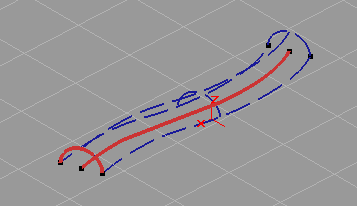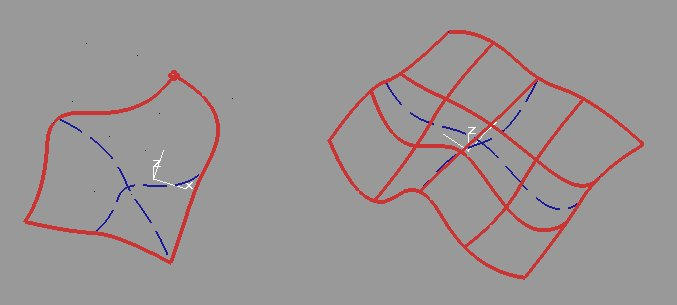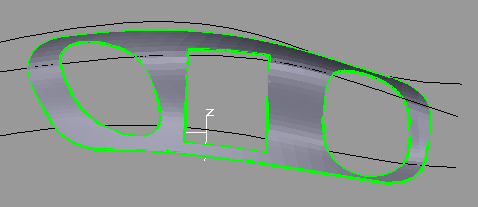```

____________________________________________________________________________

Format Specification: gCAD3D Codes for surfaces:
____________________________________________________________________________

Identification letter is A ("Area")
____________________________________________________________________________

Unlimited surfaces (support-surfaces):
Spherical surface
Revolution surface
Extrusion surface
Sweep surface
Loft surface

Trimmed, perforated surfaces:
Planar surface
Supported surface
Hatched surface

Surfaces for mockup models (tesselated surfaces):
Fan area
Strip Area

Basic functions:
Point-surface
B-spline surface

OBSOLETE:
Cylindrical surface
Ruled surface

____________________________________________________________________________

Sweep surface:

Sweep surface defined by a contour moving along a path;
eg a pipe from moving a circle along a line.
The curve is normal to the path along the whole path.

A = SWEEP contour path
contour:  curve (eg circle for a pipe)
path:     vector or curve (contour moves along path)____________________________________________________________________________

Loft surface:

Loft surface from curves across surface
A = BSP curves_across

Curves can be point, line, circle, ellipsis, spline.
2 Curves are connected with lines.
More than 2 curves are connected with splines.

Examples LOFT curves_across:Loft surface from curves along and across
A = BSP U(curves_across) U(curves_along)

Curves can be point, line, circle, ellipsis, spline.

Examples LOFT along and across:____________________________________________________________________________

Extrusion surface:

Surface from contour and extrusion vector.

A = SRU contour extrusion-vector

Examples SRU (ruled surface):
L20=P(0 0 0) P(0 0 200)
A20=SRU L20 D(100 0 0)____________________________________________________________________________

Planar surface:

Planar surface, trimmed, perforated:

A = outer-boundary [inner-boundaries ...]

Boundary curve, islands: a circle, an ellipse, a closed B-spline or
a closed CCV (CCV S = ..).

# Example Planar surface:
P20 = P(-120 -160)
# The boundary curve:
S20 = CCV P20 P(233 -186) P(223 -95) P(104 -81) P(135 134) P(-122 162) P20
# The planar surface:
A20 = S20

# Circular area with island
C29 = P(0 0 0) VAL(12)
C28 = P(0 0 0) VAL(6)
A20 = C28 C29

____________________________________________________________________________

OBSOLETE - use loft-surface (2 curves) or extrusion-surface (curve+vector)

Standard formats ruled surface:

Ruled Surface of two basic elements (PT/LN/AC/CV)

A# = SRU Object1 obj2

L20 = P(0 -10 20) P(0 10 20)
C20 ARC = P(0 -10) P(0 10) P(0 0)
A20 = SRU C20 L20

Ruled Surface of a basic element and vector:
L30 = P(0 -10 20) P(0 10 20)
D30 = D(10 10 60)
A30 = SRU L30 D30

____________________________________________________________________________

OBSOLETE - use revolved-surface or extrusion-surface

Cylindrical surface:

A = CYL Axis Radius rotation-angle1 rotation-angle2 height1 height2
Axis line or circle or plane or PT+PT or PT+LN or PT+VC
rotation-angle1 key in start angle (Default = 0) or point.
rotation-angle2 key in end angle (Default = 360) or point.
height1 key in height (from position; Default = 0) or point.
height2 key in height (from position), or point.

R22 = P(105
129.2 0) D(1 1 1)
A22 = CYL R22 12 0 360 0 30

____________________________________________________________________________

Spherical surface:

A = SPH axis radius [rotation_angle1 rotation_angle2 [height_angle1 height_angle2]]
Spherical surface.
Axis: line or circle or plane or PT+PT or PT+LN or PT+VC
rotation_angle1: key in start angle (Default = 0) or point.
rotation_angle2: key in end angle (Default = 360) or point.
height_angle1: key in start-angle (Def = 0) or point.
height_angle2: key in end-angle (Def = 180) or point.
height-avlues: 0 - 180; centerline=90 deg.

Examples:
P20 = P(68 68.9 0)
A20 = R(P20) 12

A21=SPH R(DZ) 500 0 90 90 180____________________________________________________________________________

Revolution surface:

A = SRV axis contour [rotation_angle1 rotation_angle2 [height1 height2]]
Axis            line or plane or PT+PT or PT+LN or PT+VC
Contour         line, circle or B-spline.
Contour is along axis (normal to axis: use sweep)
rotation_angle1 key in start angle (Default = 0) or point.
rotation_angle2 key in end angle (Default = 360) or point.
height1         key in startup parameter (Default = 0), or point.
height2         key in end parameter (Default = 1), or point.

The starting point of the contour element is in position angle 0 degrees;
the rotation direction is CCW.

Examples:

# Torus
P20=P(0 0 0)
P21=P(100 0 0)
L20=P20 DZ
# Vector = crossproduct from 2 lines
D20=L20 D(P21 P20)
C20=P21 25 D20
A20=SRV L20 C20 0 90 0 1

L20 = P(20 0) P(20 20)
L21 = P(50 0) P(40 20)
A20 = SRV L20 L21 270 0____________________________________________________________________________

B-spline surface:

A# = BSP, pt1Nr, pt2Nr, degree1, degree2, controlpoints, knotvector1, knotvector2
pt1Nr = number of control points in direction 1
pt2Nr = number of control points in direction 2
degree1 = degree of B-spline in direction 1
controlpoints: the control points, number = pt1Nr * pt2Nr,) 3 values (X/Y/Z.
knotvector1: the spacing parameters for direction 1; Nr = Pt1Nr + degree1 + 1

____________________________________________________________________________

Supported Surface:

Trimmed, perforated surface on (unlimited) support-surface.
Support-surface must be provided,
outer boundary and inner boundaries can be added.

A = FSUB supportsurface outer-boundary [inner-boundaries ...]

Supporting surface:
a supporting surface is always required.
All unlimited surfaces can be used.

Boundary curve, Islands:
a circle, an ellipse, a closed B-spline or a closed CCV (S = CCV ..).
The boundary curve must be located on the support surface.
If the outer contour is identical to the boundary of the support surface,
then the supporting surface can be specified as a boundary curve.

A = FSUB A S     // support surface and outer-boundary
A = FSUB A S S   // support surface, outer-boundary, 1 inner-boundary
A = FSUB A S S S // support surface, outer-boundary, 2 inner-boundaries
A = FSUB A A S   // supporting surface is not trimmed, 1 inner-boundary

# Example cone surface:
P20 = P(100 0 0)
P21 = P(200 0 0)
P22 = P20 Y(120)
P23 = P21 Y(60)
P24 = P20 P22 ANG(135) DX
P25 = P21 P23 ANG(135) dx
C20 = ARC P23 P25 P21 dx
C21 = P22 P24 P20 ARC dx
#
B20 = CON P20 P21 VAL(P22 P20) VAL(P23 P21)
# The boundary curve:
S20 = CCV P23 P22 C21 P24 P25 C20 P23
# The cone surface:
A20 = FSUB B20 S20
#____________________________________________________________________________

Fan surface

Near-circular surface of 3-n points;
All rays radiate from the first point (eg center of the circle).

A# = RCIR, P#, P#, P# <, P#>

P20 = P(5, -2)
P21 = P(9, -1)
P22 = P(8, 3)
A20 = RCIR, P20, P21, P22, P(4 3)

____________________________________________________________________________

Strip surface

Strip-like surface made of 1-n strips.

A# = RSTRIP PTNR P# P# P# <, P#>
Each strip consists of two rows of (PtNr) points
Minimum number of points is 2 * PTNR.
For 2 strips (3 * PTNR) points are required.

P20 = P(2 0)
P21 = P(4 0)
P22 = P(6 0)
P23 = P(8 0)

P30 = P(2 0 5)
P31 = P(4 1 5)
P32 = P(6 1 5)
P33 = P(8 0 5)

A21 = RSTRIP, 4, P20, P21, P22, P23, P30, P31, P32, P33

____________________________________________________________________________

Hatched surface:

A# = HAT contour interval direction
Direction = Hatching direction in degrees

P20 = P(-500 500)
S21 = CCV P20 P(400 900) P(400 300) P20
A20 = HAT S21 VAL(50) VAL(45)

Currently, no import / export - function for hatching.
Currently, no function for perforated hatching.

____________________________________________________________________________
Modifications:
2014-01-17 Loft-surface from B-spline-surface;
Ruled-surface (2 curves) added to Loft-surface
Sweep-surface new